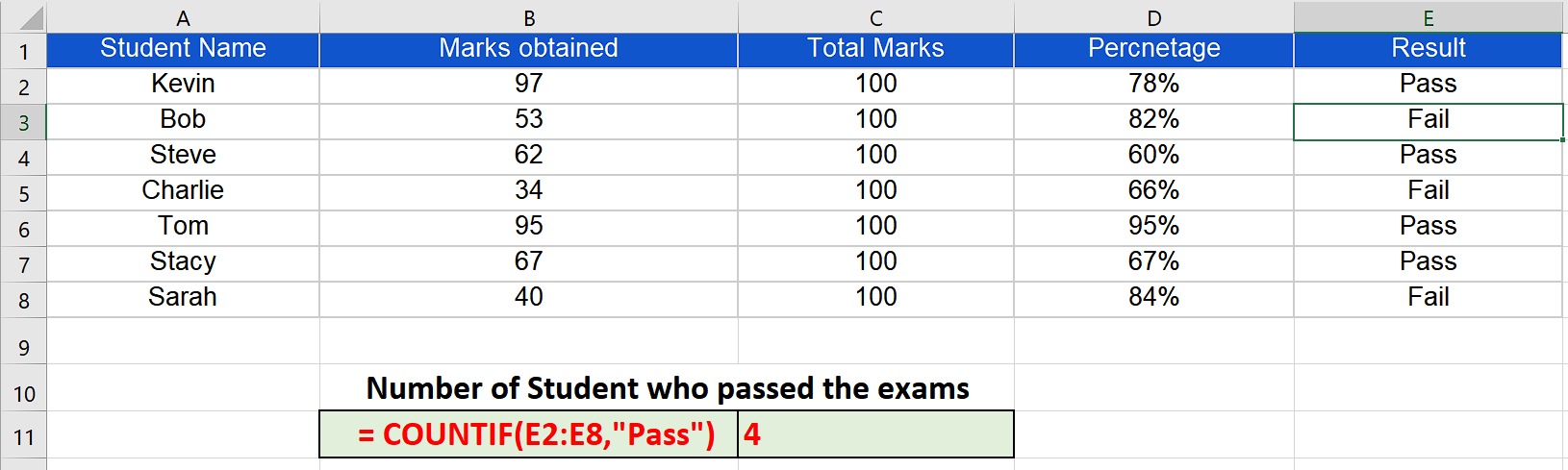# How to use Countif to count the rows containing specific word in Excel

## Structure of COUNTIF

The standard syntax of countif function in excel is shown below;

= COUNTIF(range,criteria)

• range (where to look in for something)

A range can be a single column with multiple rows or it could consist of multiple columns and multiple rows. For example “A1:B10”. This will tell the formula to search through two columns A & B till the 10th row.

• criteria (what to look for)

Criteria will be a special logical test which will depend upon the situation and the nature of values that we are looking for. In our example we are looking for all non-blank cells so we will use a special logical condition in the formula which will tell Excel to count only those cells which contain a specific keyword.

Implementing the Formula on an Actual Data Set

Let’s take an example data set and find out the number of cells which contain a specific word “Pass” in it.

We can see in the example data set that it consists of information about marks obtained by students, percentages and final status “Pass” or “Fail”. We wish to find out how many students have passed the exam so we’ll use Excel’s built-in formulas to find a solution to our problem by following these steps;When we are working to analyze data sets in Excel, often there is a requirement to count those cells which contain a specific keyword. Excel provides some very easy ways to find out the number of cells containing a keyword within a specific range.

In this tutorial we will learn how we can use Excel’s built-in “COUNTIF” function to calculate the number of cells which contain a specific keyword. The best way to understand how countif works is to first understand the structure of the function and the parameters it requires.

### Step 1 – Implement the formula in a suitable cell:– Choose a suitable cell where you wish to implement the formula.

– Use the following formula in that cell and press enter key.

= COUNTIF(E2:E8,”Pass”)

– The desired result will be displayed in this cell as soon as you will press the enter key as shown in the picture above.

So by using COUNTIF with a special condition to count the blank rows in column E we can find out that only 4 tasks passed the exam.

## Breakdown of the Logical Condition used inside the formula

We used the formula = COUNTIF(E2:E8,”Pass”). Let’s see how we told Excel where to look and what to look for.

• The first parameter E2:E8 tells Excel that the data range in which we are going to look for something is in column E and from row 2 to row 8 only.
• The second parameter was “Pass”. It represents a logical condition which tells Excel to look for only those cells which have the specific keyword “Pass”. When Excel finds a cell with the keyword “Pass” in it. The counter is increased. Our data set had four cells in that range which had the keyword “Pass” so the result was 4 as desired.